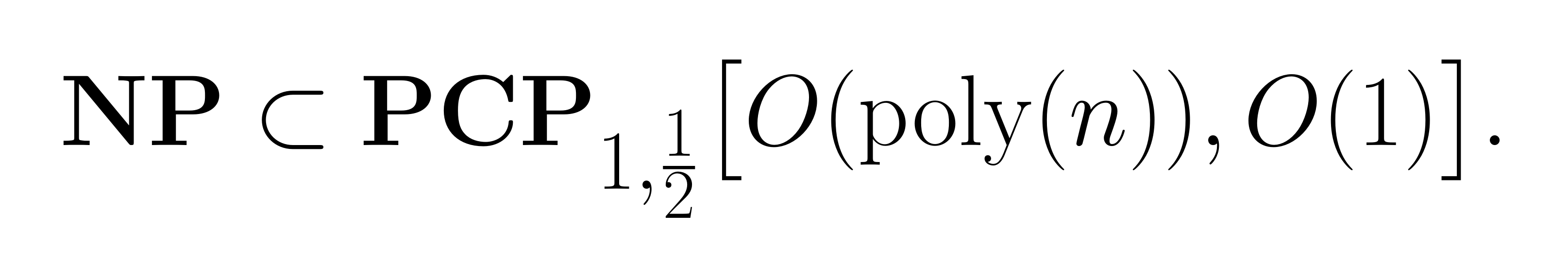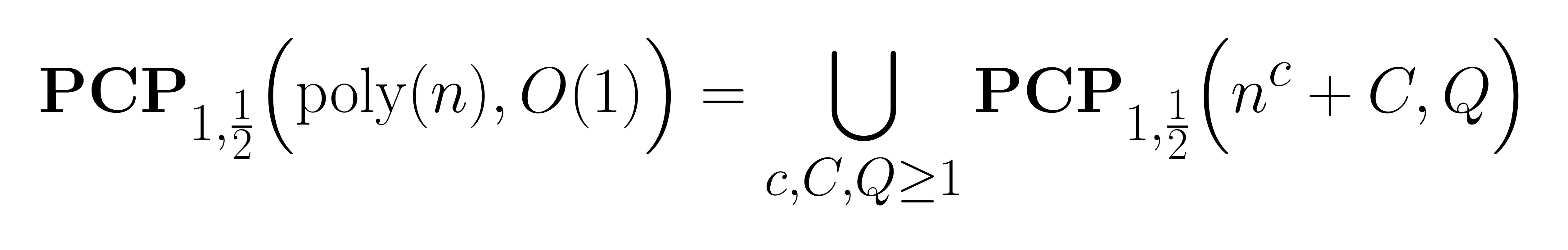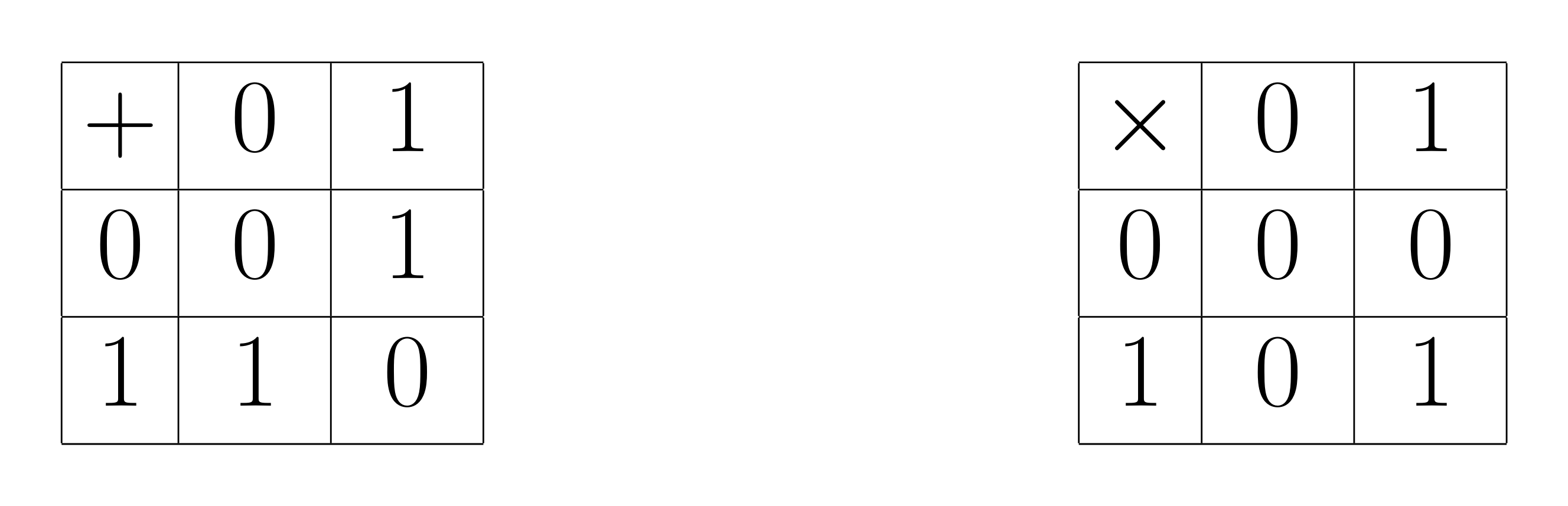# 1 Recap

In the first post in this series we gave basic definitions : the complexity classes NP and PCP and what it takes for a decision problem to have probabilistically checkable proofs (PCPs), and thus lie in a PCP complexity class. We also teased the statement of the weak PCP theorem:whereIn this second post we sketch a proof of this result. We warn the reader that proving it requires a bit of linear algebra and (unsurprisingly) some basic probability theory. But the general gist of the proof isn’t all that complicated (although it is ingenious). As is usual in these kinds of proofs, the hard part is establishing soundness. The proof is taken from this excellent Introduction to the PCP theorem by Min Jae Song.

To simplify notations, write PCP for the complexity class PCP$$_{1,\frac12}(O(\ln(n)), 0(1))$$ that appears in the statement of the strong PCP theorem and wPCP for the complexity class PCP$$_{1,\frac12}(\mathrm{poly}(n), 0(1))$$ that appears in the statement of the weak PCP theorem.

There will be a lot of ground to cover, so we shall divide the work up into smaller chunks and provide the reader with a bird eye’s view of what’s to come.

## 2.1 Preprocessing

The bulk of this post will be about constructing PCPs for 3-SAT using the decision problem known as QUADEQ as an intermediary. We will thus see how an to reduce 3-SAT to QUADEQ (along the way we shall of course describe QUADEQ, i.e. its instances and witnesses). This first transformation from 3-SAT to QUADEQ is very lean: hardly any complexity gets added. In doing so, we move from a typical computer science problem to one that has more of a linear algebra flavour to it.

The next step is again to change the problem to an equivalent one, however, this transformation comes at a tremendous cost: the solution/witness space grows exponentially larger in size. In other words, this modification brutally impacts the witness / PCP proof-format size for QUADEQ.

## 2.2 The Prover’s job and the PCP proof format

Thankfully, this proof-format is quite concrete and explicit: rather than a 0/1-assignment as in 3-SAT or a $$\{0, 1\}$$-vector for QUADEQ, proofs in the PCP format are pairs $$(f,g)$$ of $$\{0, 1\}$$-valued functions given by their values $$\Gamma_f$$ and $$\Gamma_g$$. When we say that a function $$f$$ is given by its values, what we mean is that we are given a complete description of all its values. For instance, we might describe an integer valued map $$f:\{-5,-3,-2,-1,0,4\}\to\mathbb{N}$$ as a list of tuples, say $\Gamma_f=[(-5,26), (-3,10), (-2,5), (-1,2), (0,1), (4,17)]$ where each tuple $$(x,y)\in\Gamma_f$$ represents an input value $$x$$ of $$f$$, and the output value $$y=f(x)$$. This is to be contrasted with algorithmic definitions of functions, where functions are described by means of a recipe that allows one to compute their values for different inputs, say $f:\left\{ \begin{array}{rcl} \mathscr{D}_f & \longrightarrow & \mathbb{N}\\ x & \mapsto & x^2+1 \end{array} \right.$ with domain $$\mathscr{D}_f=\{-5,-3,-2,-1,0,4\}$$.

Returning to our discussion of the PCP proof format, correct proofs are special pairs of functions (linear functions) (Walsh-Hadamard codes) that are derived from a witness. The series of conversions

$(\mathbf{3}\textbf{-}\mathbf{SAT}\text{-witness}) \Rightarrow (\mathbf{QUADEQ}\text{-witness}) \Rightarrow (\mathbf{QUADEQ}\text{ PCP proof})$

is handled by the Prover and involves only trivial computations (dot products), albeit a great many of them. It requires as only input a witness $$u$$ of the specific 3-SAT instance, and cannot be precomputed. In fact, it involves so many computations that producing such PCP proofs for instances of 3-SAT with 10 Boolean variables already is totally out of the question. We stress that this is not meant to be a practical PCP scheme. At this point we will have described the proof format, and how a Prover contructs a proof $$(\Gamma_f, \Gamma_g)$$ from witness data $$u$$.

## 2.3 The Verifier’s job

The job of the Verifier is very easy. It applies three different tests, all of which are instances of a single simple master test. To behold, the Verifier is handed a “proof” $$\pi=(\Gamma_f,\Gamma_g)$$, and its job is

1. to toss a polynomial amount of random coins,
2. to perform simple polynomial time computations using only bits, and outputting either 1 or 0.

The purpose of the random coin tosses is to come up with random locations within the domains of the maps $$\Gamma_f$$ and $$\Gamma_g$$ where to perform queries. Thus the Verifier reads a constant number of randomly (and independently) sampled bits ($$a,b,c,d,e,A,B,C,D,E,F,G$$ below) from the proof $$(\Gamma_f, \Gamma_g)$$ and uses them as inputs for computations. To give the reader an idea of just how simple the actual computations are, we list them here:

1. fetch $$a,b,c\longleftarrow\Gamma_{f}$$, check if $$a+b\overset?=c$$;
2. fetch $$A,B,C\longleftarrow\Gamma_{g}$$, check if $$A+B\overset?=C$$;
3. fetch $$d,e\longleftarrow\Gamma_{f}$$ and $$D,E\longleftarrow\Gamma_{g}$$ and check $$d\cdot e\overset?=D+E$$;
4. fetch $$F,G\longleftarrow\Gamma_{g}$$ and $$\vec{x}\longleftarrow\mathbb{F}_2^m$$, compute a dot product $$p=\langle \vec{b}\mid \vec{x}\rangle$$ check and if $$F+G\overset?=p$$.

(In the last point, $$\vec{b}$$ is a vector that is part of the problem description) Of course there is some structure to these queries which we gloss over for now, but we insist on the fact that the actual computations are incredibly tame. To be precise, operations of type 1. , 2., 3. and the check of 4. are constant time operations on bits’’ $$a, b, c, d, e, A, B, C, D, E, F, G \in\{0,1\}$$. Computing the dot product $$\langle \vec{b}\mid \vec{x}\rangle$$ has linear complexity in the vector size.

This whole ordeal is repeated a $$Q$$ times (a few hundred times, say), and if all tests pass, the Verifier outputs $$\texttt{Accept}$$.

## 2.4 Testing and Soundness

Good “tests” are the central pillar of the PCP Verifier. One might claim, somewhat facetiously, that we really only use the previously mentioned simple-minded master test. All the subtlety resides in how to use it. Making good use of some properties of linear and bilinear maps is front and center in this respect. The whole point of the very expensive transformation alluded to earlier is so that we may use these. The core benefit we extract is that this new proof format brings uniform separation to the solution space where previously potential solutions could be arbitrarily close.

It will be quite clear from that construction that proofs which were correctly constructed by an honest Verifier in possession of correct witness data have an acceptance rate of 100%, so Completeness is a given. Where things get interesting is with Soundness, i.e. in showing that a dishonest Prover can’t come up with fake proofs for NO instances of QUADEQ that would get accepted $$\geq 50\%$$ of the time. This requires some real ingenuity, and this is when the testing we talked about earlier becomes important. Careful analysis of this protocol shows that this Verifier has the soundness required of a weak PCP Verifier, and so 3-SAT has PCPs. But 3-SAT is but one decision problem in the infinite collection of decision problems that is NP : do we have to start all over for other decision problems in NP? No if wPCP contains one NP complete problem, then it contains all of NP. Since 3-SAT (and QUADEQ for that matter) is NP-complete, the weak PCP theorem is proven :)

# 3 Algebraisation of 3-SAT: from 3-SAT to QUADEQ

We describe how to convert an instance of 3-SAT, a decision problem from the realm of Boolean algebra and Boolean constraint systems, into an equivalent problem formulated in terms of linear algebra over the field with two elements $$\mathbb{F}_2$$.

## 3.1 Algebra over the field $$\mathbb{F}_2$$.

All the algebra will happen over the field with two elements $$\mathbb{F}_2$$. We quickly recall its arithmetic. As a set $$\mathbb{F}_2=\{0,1\}$$. Addition and multiplication are defined byNote that for all $$x\in\mathbb{F}_2$$, $$x^2=x$$. The arithmetic of $$\mathbb{F}_2$$ is that of the usual integers if all we care about is parity, i.e. even vs. odd numbers. Indeed, we can pass from the integers $$\mathbb{Z}$$ to $$\mathbb{F}_2$$ by sending an arbitrary integer $$n\in\mathbb{Z}$$ to $$p(n) = 0$$ if $$n$$ is even, and to $$p(n) = 1$$ if $$n$$ is odd. Then, since the sum of two integers is even iff the two integers have the same parity and the product of two integers is odd iff both are odd, we see that for arbitrary integers $$n,m$$

$p(n+m) = p(n) + p(m) \quad\text{and}\quad p(n\times m) = p(n) \times p(m)$

where the symbols $$+$$ and $$\times$$ on the right-hand side of each equation are those of $$\mathbb{F}_2$$.

## 3.2 Boolean algebra from the $$\mathbb{F}_2$$-point of view

We can express all Boolean operations by using the field operations $$+$$ and $$\times$$ of $$\mathbb{F}_2$$.

The correspondence between basic Boolean operations and algebraic operations is contained in the following table: